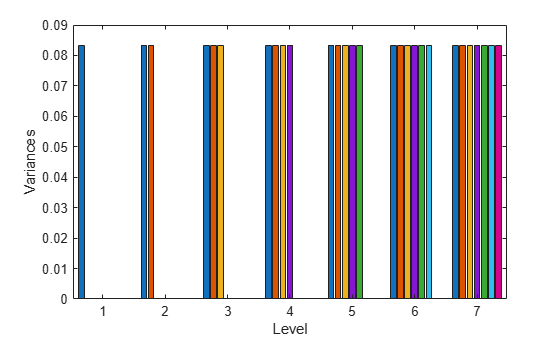# reduceDimensions

Reduce dimensions of Sobol point set

## Syntax

``pr = reduceDimensions(p,d)``

## Description

example

````pr = reduceDimensions(p,d)` reduces the Sobol quasirandom point set `p` to the first `d` dimensions. `d` must be less than or equal to the number of dimensions in `p`.The reduced point set `pr` is a `sobolset` object.```

## Examples

collapse all

Generate a seven-dimensional Sobol point set and scramble the points.

```p = sobolset(7); ps = scramble(p,'MatousekAffineOwen')```
```ps = Sobol point set in 7 dimensions (9007199254740992 points) Properties: Skip : 0 Leap : 0 ScrambleMethod : MatousekAffineOwen PointOrder : standard ```

Split the first 7168 points in `ps` into seven levels of 1024 points each. Reduce the first 1024 points to be one-dimensional, the second 1024 points to be two-dimensional, and so on. For each level, compute the variance of the point values in each dimension.

```variance = NaN(7); for level = 1:7 pr = reduceDimensions(ps,level); pr.Skip = (level-1)*1024; pts = pr(1:1024,:); variance(level,1:level) = var(pts); end```

Plot the variances. The dark blue bars show the variance of the points in the first dimension, the dark orange bars show the variance of the points in the second dimension, and so on.

```bar(variance) xlabel('Level') ylabel('Variances')```## Input Arguments

collapse all

Sobol point set, specified as a `sobolset` object.

Number of dimensions to retain from the point set `p`, specified as a positive integer scalar between 1 and the number of dimensions in `p`. The function always retains the first `d` dimensions of `p`.

Data Types: `single` | `double`

## Version History

Introduced in R2019a